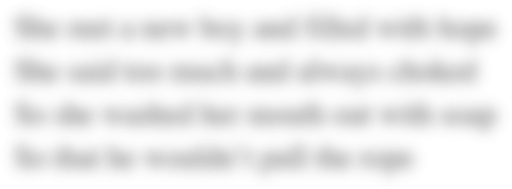# MAT 540 Week 8 Assignment - Julia's Food Booth

Assignment 1. Linear Programming Case Study
Your instructor will assign a linear programming project for this assignment according to the following specifications.
It will be a problem with at least three (3) constraints and at least two (2) decision variables. The problem will be bounded and feasible. It will also have a single optimum solution (in other words, it won’t have alternate optimal solutions). The problem will also include a component that involves sensitivity analysis and the use of the shadow price.
You will be turning in two (2) deliverables, a short writeup of the project and the spreadsheet showing your work.
Writeup.
Your writeup should introduce your solution to the project by describing the problem. Correctly identify what type of problem this is. For example, you should note if the problem is a maximization or minimization problem, as well as identify the resources that constrain the solution. Identify each variable and explain the criteria involved in setting up the model. This should be encapsulated in one (1) or two (2) succinct paragraphs.
After the introductory paragraph, write out the L.P. model for the problem. Include the objective function and all constraints, including any non-negativity constraints. Then, you should present the optimal solution, based on your work in Excel. Explain what the results mean.
Finally, write a paragraph addressing the part of the problem pertaining to sensitivity analysis and shadow price.
Excel.
As previously noted, please set up your problem in Excel and find the solution using Solver. Clearly label the cells in your spreadsheet. You will turn in the entire spreadsheet, showing the setup of the model, and the results.
Click here to view the grading rubric for this assignment.
Complete the "Julia's Food Booth"case problem on page 109 of the text. Address each of the issues A-D according the instructions given.
Formulate and solve an L.P. model for this case.
Evaluate the prospect of borrowing money before the first game.
Evaluate the prospect of paying a friend \$100/game to assist.
Analyze the impact of uncertainties on the model.
Week 8 Assignment 1:
Assignment #1: Case Problem "Julia's Food Booth"
Complete the "Julia's Food Booth" case problem on page 109 of the text. Address each of the issues A - D according the instructions given.
·         (A) Formulate and solve an L.P. model for this case.
·         (B) Evaluate the prospect of borrowing money before the first game.
·         (C) Evaluate the prospect of paying a friend \$100/game to assist.
·         (D) Analyze the impact of uncertainties on the model.
• Write up.
Your write up should introduce your solution to the project by describing the problem. Correctly identify what type of problem this is. For example, you should note if the problem is a maximization or minimization problem, as well as identify the resources that constrain the solution. Identify each variable and explain the criteria involved in setting up the model. This should be encapsulated in one (1) or two (2) succinct paragraphs.
After the introductory paragraph, write out the L.P. model for the problem. Include the objective function and all constraints, including any non-negativity constraints. Then, you should present the optimal solution, based on your work in Excel. Explain what the results mean.
Finally, write a paragraph addressing the part of the problem pertaining to sensitivity analysis and shadow price.
Field of study:
Date Due:
Tuesday, October 23, 2018